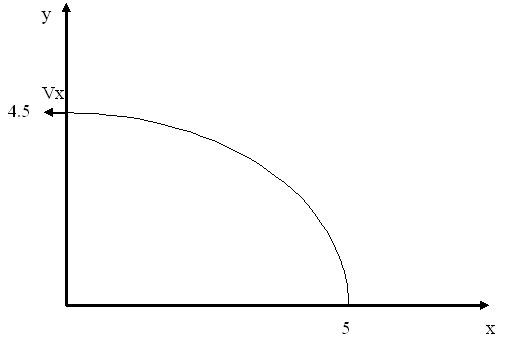# Answer to Question #4450 in Mechanics | Relativity for archana

Question #4450
Romeo is chuckling pebbles gently up to Juliets window, and he wants the pebbles to hit the window with only a horizontal component of velocity. He is standing at the edge of a rose garden 4.5 m below her window and 5.0 m from the base of the wall. How fast are the pebbles going when they hit her window?
1
2011-10-11T08:29:19-0400Suppose the pebble had initial velocities Vx and Vy (V=sqrt(Vx^2+Vy^2)). As pebbles hit the window with only a horizontal component of velocity Vx, so
the vertical component becomes equal to zero while the pebble is in the air.
Let&rsquo;s find this time t from the equation for vertical displacement:
H = 4.5 = (gt^2)/2 =&gt; t = 3/sqrt(g).
The equation for horizontal displacement is:
S = 5 = Vx*t =&gt; Vx = 5/t = 5*sqrt(g)/3 = 5*sqrt(9.8)/3 = 5.22 m/s.
So, Vx = 5.22 m/s.

Need a fast expert's response?

Submit order

and get a quick answer at the best price

for any assignment or question with DETAILED EXPLANATIONS!